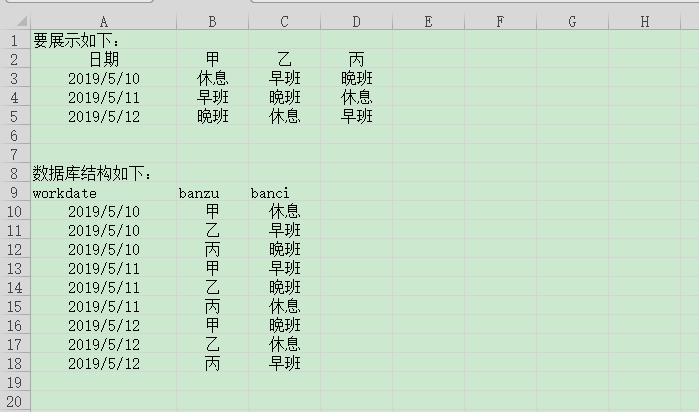oracle 怎么用SQL语句实现上面的表展示，求各位朋友指点。注意：甲乙丙这个班在数据库不是固定的，有空能是别的名称。

3个回答

select* from table_test
pivot(max(banci) for banzu in('甲' 甲,'乙' 乙,'丙' 丙))

in 里面传进来你要的班组

``````select workdate 日期,case when banzu = '甲' then banci end 甲,case when banzu = '乙' then banci end 乙,case when banzu = '丙' then banci end 丙 from table_name group by  workdate;
``````wuronghong 回复have_power: 指点下，怎么弄？have_power 回复wuronghong: 那你这得用存储过程来实现，简单的sql肯定是不行了。最怕的就是这种一次性把数据介绍不清楚的。wuronghong 这个甲乙丙不是固定的，应该要用到游标

``````select a.workdate as 日期 ,a.banci as 甲,b.banci as 乙,c.banci as 丙 from(select workdate ,banci from question2 where banzu='甲') as a INNER JOIN
(select workdate ,banci from question2 where banzu='乙') b on a.workdate=b.workdate INNER JOIN
(select workdate ,banci from question2 where banzu='丙') as c on a.workdate=c.workdate
``````

``````select workdate 日期,Max(case when banzu = '甲' then banci end) 甲,Max(case when banzu = '乙' then banci end )乙,Max(case when banzu = '丙' then banci end )丙 from question2 group by  workdate;
``````

## 我都是用mysql数据库测试的，如果答案没错的话希望采纳一楼